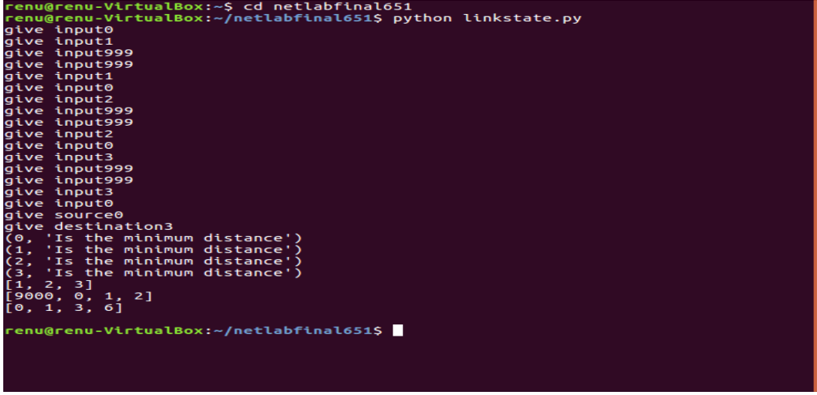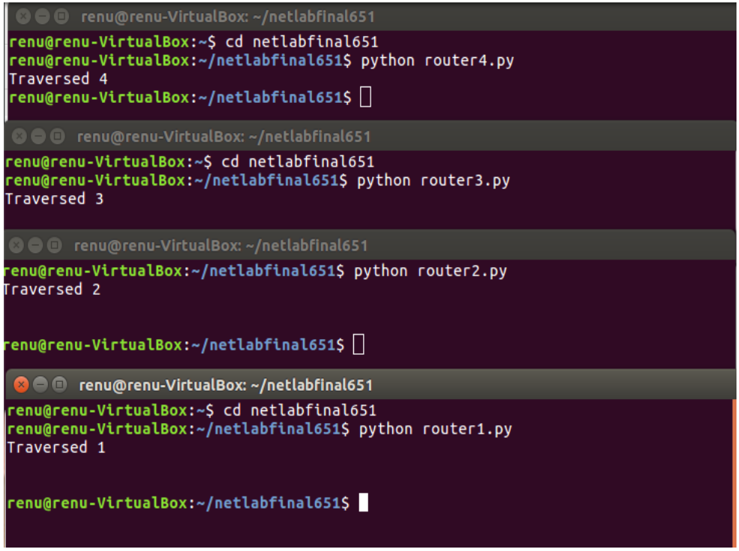Open in App
Not now

# Shortest Path Problem Between Routing Terminals – Implementation in Python

• Difficulty Level : Expert
• Last Updated : 27 Feb, 2020

The famous Dijkstra’s algorithm can be used in a variety of contexts – including as a means to find the shortest route between two routers, also known as Link state routing. This article explains a simulation of Dijkstra’s algorithm in which the nodes (routers) are terminals.
Once the shortest path between two nodes (terminals) is calculated, the shortest path itself is sent as a message to each terminal on its path in sequence, until the destination terminal is reached. Whenever the message has traversed a node, its terminal displays the traversal. In this way, it is possible to both see and simulate the passage of a message across a shortest calculated route.

The procedure to run the following code is as follows:

• Execute the driver code
• Before providing any input to the driver code, run the router codes router1.py, router2.py, etc. in separate terminals/tabs.
• Now provide input to the driver code in the form of a matrix G, in which any entry `G[i, j]` is the distance from node i to node j. The matrix must be symmetrical. If i=j, then` D[i, j]=0` as the distance between a node and itself is considered to be nothing. If there is no direct connection between two nodes, then `D[i, j]=999` (the equivalent of infinity).
• Specify source and destination nodes, where the nodes vary from 0 to 3, and represent terminals 1 to 4 respectively.

This implementation specifies four nodes but this can easily be extended to N nodes with N terminals and port numbers representing processes running on them respectively.

Consider the following example of a four-node network, with distances between them as specified and nodes numbered 0 to 3 from left to right:Distances between terminals

For this network, the matrix G with entries G[i, j] as specified above would be:

```[[0, 1, 999, 999],
[1, 0, 2, 999],
[999, 2, 0, 3],
[999, 999, 3, 0]]
```

This matrix would have to be input to the driver code. Dijkstra’s algorithm is used to find the shortest path between source and destination. A list containing the remaining path is sent to each node en route to the final destination.

The implementation in Python is specified below.

 `# Driver Code for implementing Dijkstra's algorithm``import` `socket``import` `sys``import` `pickle`` ` `S ``=` `set``() ``G ``=``[] ``# adjacency matrix`` ` `# give input matrix``for` `i ``in` `range``(``4``): ``    ``listo ``=``[``0``, ``0``, ``0``, ``0``]``     ` `    ``for` `j ``in` `range``(``4``):``        ``listo[j]``=` `int``(``input``(``"give input"``))``    ``G.append(listo)``     ` `source ``=` `int``(``input``(``"give source"``)) ``destination ``=` `int``(``input``(``"give destination"``)) ``Q ``=``[] ``# empty queue`` ` `for` `i ``in` `range``(``4``):``    ``Q.append(i)``     ` `d ``=``[``0``, ``0``, ``0``, ``0``] ``# initialize d values``pi ``=``[``0``, ``0``, ``0``, ``0``] ``# initialize pi values`` ` `for` `i ``in` `range``(``4``):``    ``if``(i ``=``=` `source):``        ``d[i]``=` `0``    ``else``:``        ``d[i]``=` `999``for` `i ``in` `range``(``4``):``    ``pi[i]``=` `9000``S.add(source)`` ` `# While items still exist in Q``while` `(``len``(Q)!``=` `0``): ``     ` `    ``# Find the minimum distance x from``    ``# source of all nodes in Q``    ``x ``=` `min``(d[q] ``for` `q ``in` `Q) ``    ``u ``=` `0``    ``for` `q ``in` `Q:``        ``if``(d[q]``=``=` `x):``             ` `            ``# Find the node u in Q with minimum ``            ``# distance x from source ``            ``u ``=` `q ``             ` `    ``print``(u, ``"Is the minimum distance"``)``    ``Q.remove(u) ``# removed the minimum vertex``    ``S.add(u)``    ``adj ``=``[]``    ``for` `y ``in` `range``(``4``):``         ` `        ``# find adjacent vertices to minimum vertex``        ``if``(y !``=` `u ``and` `G[u][y]!``=` `999``):     ``            ``adj.append(y)``             ` `     ``# For each adjacent vertex, perform the update``     ``# of distance and pi vectors        ``    ``for` `v ``in` `adj:        ``        ``if``(d[v]>(d[u]``+``G[u][v])):``            ``d[v]``=` `d[u]``+``G[u][v] ``            ``pi[v]``=` `u ``# update adjacents distance and pi``route ``=``[]``x ``=` `destination`` ` `# If destination is source, then pi[x]= 9000. ``if``(pi[x]``=``=` `9000``): ``    ``print``(source)``else``:``     ` `    ``# Find the path from destination to source``    ``while``(pi[x]!``=` `9000``): ``        ``route.append(x)``        ``x ``=` `pi[x]``    ``route.reverse() ``     ` `     ` `print``(route) ``# Display the route``print``(pi) ``# Display the path vector``print``(d) ``# Display the distance of each node from source`` ` `'''We will now send the calculated minimal route to the terminal ``# representing 'source'. From the source terminal, the 'route' list ``# will be sent to the next hop en route to the final destination. `` ` `# At each intermediate terminal, the router removes its own identity `` ``from the list and sends the rest of the route to the next router. `` ``This continues until the final router is reached.'''``  ` `sendingroute ``=` `pickle.dumps(route)``sockets ``=``[``8895``, ``8896``, ``8897``, ``8898``]``sock ``=` `socket.socket(socket.AF_INET, socket.SOCK_STREAM) ``sock.connect((socket.gethostname(), sockets)) `` ` `try``:``     ` `    ``# try sendall if it doesn't work. ``    ``sock.send(sendingroute) ``finally``:``    ``print``("")``sock.close()`

 `# Code for Router 1``import` `socket``import` `sys``import` `pickle`` ` `for` `i ``in` `range``(``1``) :``    ``sock ``=` `socket.socket(socket.AF_INET, socket.SOCK_STREAM)``    ``sock.bind((socket.gethostname(), ``8895``))``    ``sock.listen(``1``)``    ``connection, client_address ``=` `sock.accept()``    ``route ``=``[]``    ``sockets ``=``[``8895``, ``8896``, ``8897``, ``8898``]`` ` `    ``while` `1``:``        ``try``:``            ``route ``=` `pickle.loads(connection.recv(``1024``))``    ``except` `EOFError:``        ``break`      `        ``finally``:``            ``break``    ``print``(``"Traversed 1"``) ``    ``socknext ``=` `socket.socket(socket.AF_INET, socket.SOCK_STREAM)`` ` `    ``if``(``len``(route)>``0``):``        ``x ``=` `route[``0``]``        ``route.remove(x)``        ``dataroute ``=` `pickle.dumps(route)``        ``socknext.connect((socket.gethostname(), sockets[x]))``        ``try``:``            ``socknext.send(dataroute) ``# try sendall``            ``data ``=` `socknext.recv(``16``)``            ``print``(data)``       ``finally``:``               ``print``("")``        ``socknext.close()`

 `# Code for Router 2``import` `socket``import` `sys``import` `pickle`` ` `for` `i ``in` `range``(``1``) :`` ` `    ``sock ``=` `socket.socket(socket.AF_INET, socket.SOCK_STREAM)``    ``sock.bind((socket.gethostname(), ``8896``))``    ``sock.listen(``1``)``    ``connection, client_address ``=` `sock.accept()``    ``route ``=``[]``    ``sockets ``=``[``8895``, ``8896``, ``8897``, ``8898``]`` ` `    ``while` `1``:``        ``try``:``            ``route ``=` `pickle.loads(connection.recv(``1024``))``    ``except` `EOFError:``        ``break`      `        ``finally``:``            ``break``    ``print``(``"Traversed 2"``)`` ` `    ``socknext ``=` `socket.socket(socket.AF_INET, socket.SOCK_STREAM)`` ` `    ``if``(``len``(route)>``0``):`` ` `    ``x ``=` `route[``0``]``        ``route.remove(x)``        ``dataroute ``=` `pickle.dumps(route)``        ``socknext.connect((socket.gethostname(), sockets[x]))``        ``try``:``            ``socknext.send(dataroute) ``# try sendall``            ``data ``=` `socknext.recv(``16``)``            ``print``(data)``       ``finally``:``               ``print``("")``        ``socknext.close()`

 `# Code for Router 3``import` `socket``import` `sys``import` `pickle`` ` `for` `i ``in` `range``(``1``) :``    ``sock ``=` `socket.socket(socket.AF_INET, socket.SOCK_STREAM)``    ``sock.bind((socket.gethostname(), ``8897``))``    ``sock.listen(``1``)``    ``connection, client_address ``=` `sock.accept()`` ` `    ``route ``=``[]``    ``sockets ``=``[``8895``, ``8896``, ``8897``, ``8898``]``    ``while` `1``:``        ``try``:``            ``route ``=` `pickle.loads(connection.recv(``1024``))``    ``except` `EOFError:``        ``break`      `        ``finally``:``            ``break``    ``print``(``"Traversed 3"``)``    ``socknext ``=` `socket.socket(socket.AF_INET, socket.SOCK_STREAM)`` ` `    ``if``(``len``(route)>``0``):``    ``x ``=` `route[``0``]``        ``route.remove(x)``        ``dataroute ``=` `pickle.dumps(route)``        ``socknext.connect((socket.gethostname(), sockets[x]))``        ``try``:``            ``socknext.send(dataroute) ``# try sendall``            ``data ``=` `socknext.recv(``16``)``            ``print``(data)``       ``finally``:``               ``print``("")``        ``socknext.close()`

 `# Code for Router 4``import` `socket``import` `sys``import` `pickle`` ` `for` `i ``in` `range``(``1``) :``    ``sock ``=` `socket.socket(socket.AF_INET, socket.SOCK_STREAM)``    ``sock.bind((socket.gethostname(), ``8898``))``    ``sock.listen(``1``)``    ``connection, client_address ``=` `sock.accept()``    ``route ``=``[]``    ``sockets ``=``[``8895``, ``8896``, ``8897``, ``8898``]`` ` `    ``while` `1``:``        ``try``:``            ``route ``=` `pickle.loads(connection.recv(``1024``))``    ``except` `EOFError:``        ``break`      `        ``finally``:``            ``break`` ` `    ``print``(``"Traversed 4"``)``    ``socknext ``=` `socket.socket(socket.AF_INET, socket.SOCK_STREAM)`` ` `    ``if``(``len``(route)>``0``):``    ``x ``=` `route[``0``]``        ``route.remove(x)``        ``dataroute ``=` `pickle.dumps(route)``        ``socknext.connect((socket.gethostname(), sockets[x]))``        ``try``:``            ``socknext.send(dataroute) ``# try sendall``            ``data ``=` `socknext.recv(``16``)``            ``print``(data)``       ``finally``:``               ``print``("")``        ``socknext.close()`Dijkstra Output

Terminal Output –Terminal Output

My Personal Notes arrow_drop_up